# Gysel even/odd mode analysis

Click here to go to our main page on Gysel's power divider

The Gysel schematic is shown below. In this case we are assuming you want a perfect 3 dB split, if you want to analyze the arbitrary power split for us be our guest... It consists of a ring that is six quarter-wave sections in circumference, much like the rat race coupler. There are five connections to it, the sixth one on the right is left as an open circuit. As a power combiner, the two ports "Input 1" and "Input 2" are where power comes in. In the schematic we have assigned three different impedances to the six sections, due to symmetry (Z1, Z2 and Z3). We have assumed that the "difference ports" are both terminated in Zdelta, but made no assumptions as to this value. We will use even and odd mode analyses to determine the value of these impedances.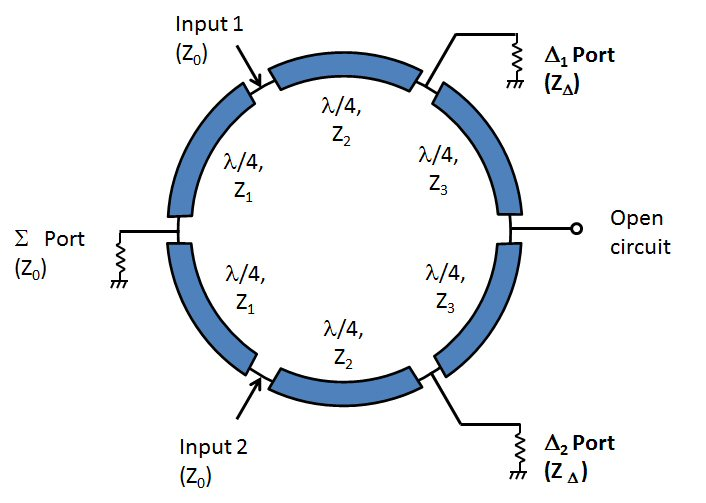## Even mode analysis

In the even mode, two signals enter the Gysel in phase at Input 1 and Input 2. In this case the nodes at the half-way points can be replaced with open circuits. Note that the sum port Z0 load is split into two loads of twice the resistance.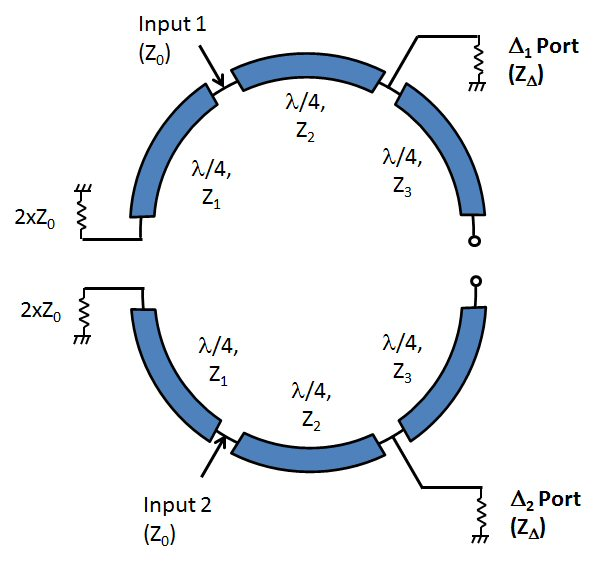On the right side, the open circuit quarter-wave stubs effectively ground the delta ports. Another quarterwave away, these ports are now open circuits, much the way a bias tee is made using stubs to choke off RF. The circuit can be simplified and we can solve for Z1. If Z0 is 50 ohms, Z1 must be 70.7 ohms.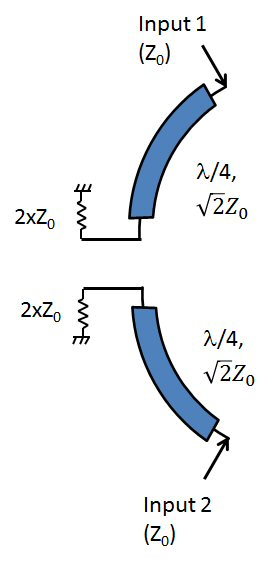## Odd mode analysis

In the odd mode the inputs are driven out of phase by 180 degrees at Input 1 and Input 2. In this case the nodes at the half-way points act as virtual short circuits, and the schematic below applies.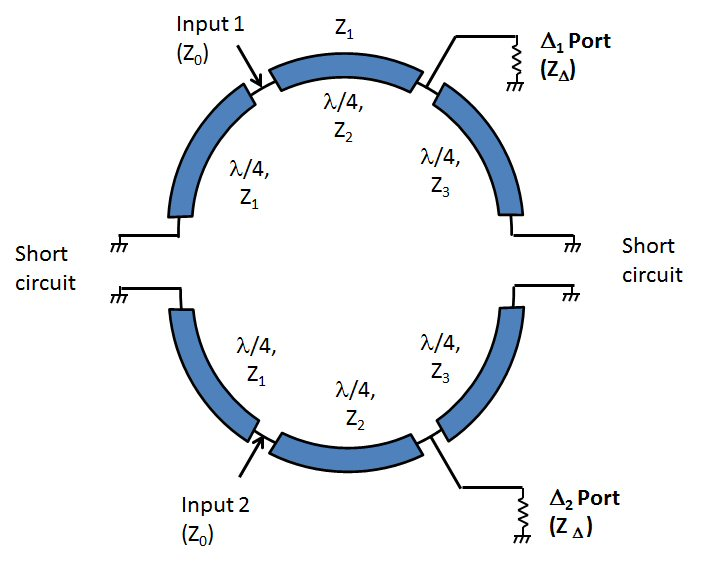The four short circuits become open circuits as they pass through four quarter-wave stubs, and we are left with the following simplification. Note that if the terminations Zdelta are 50 ohms, the Z2 sections are also 50 ohms; if the terminations are 100 ohms, Z2 is 70.7 ohms.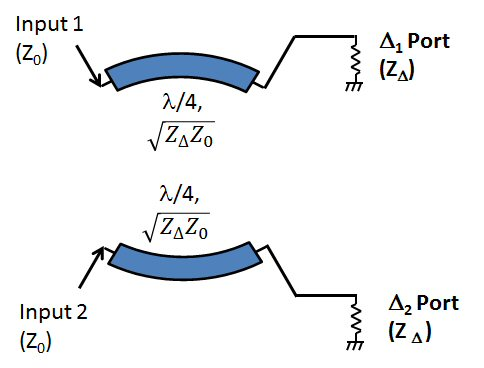That is all you can solve for. Turns out the sections Z3 are "don't care" ohms from this analysis. Typically they will want to be the lowest impedance you can achieve, for maximum bandwidth.

Below is the schematic with the impedance conditions we have identified. "Z*" means you can chose the impedance for Z3.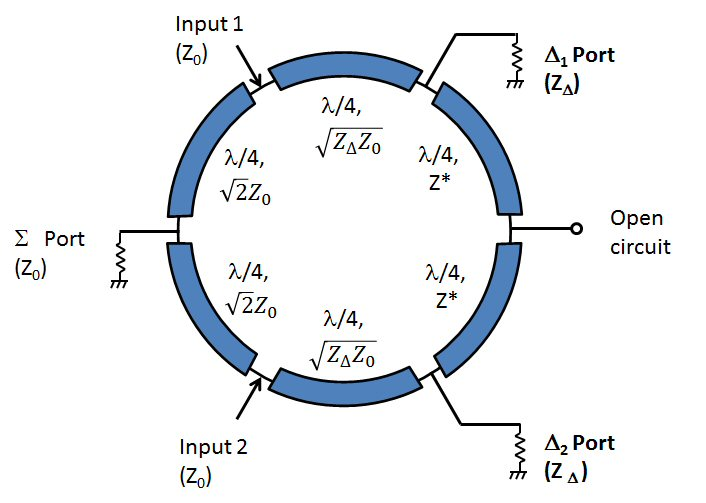If the terminations are 100 ohms, you can create a Gysel where the ring impedance is uniformly SQRT(2)xZ0, the same as the classic rat race coupler. However, only a fool would do this, you are throwing away bandwidth by NOT reducing the impedance Z3.

Check out some S-parameter plots on our main Gysel page that illustrate the degrees of freedom provided by ZT and Z3.

Here is some information on two types of unequal split Gysels, although we didn't do even-odd mode analyses for them.

Author : Unknown Editor